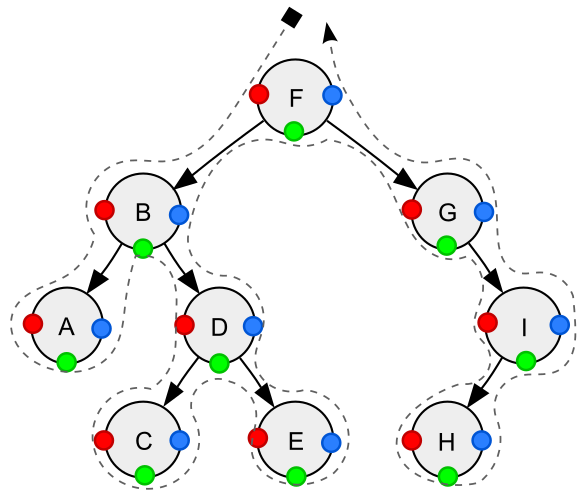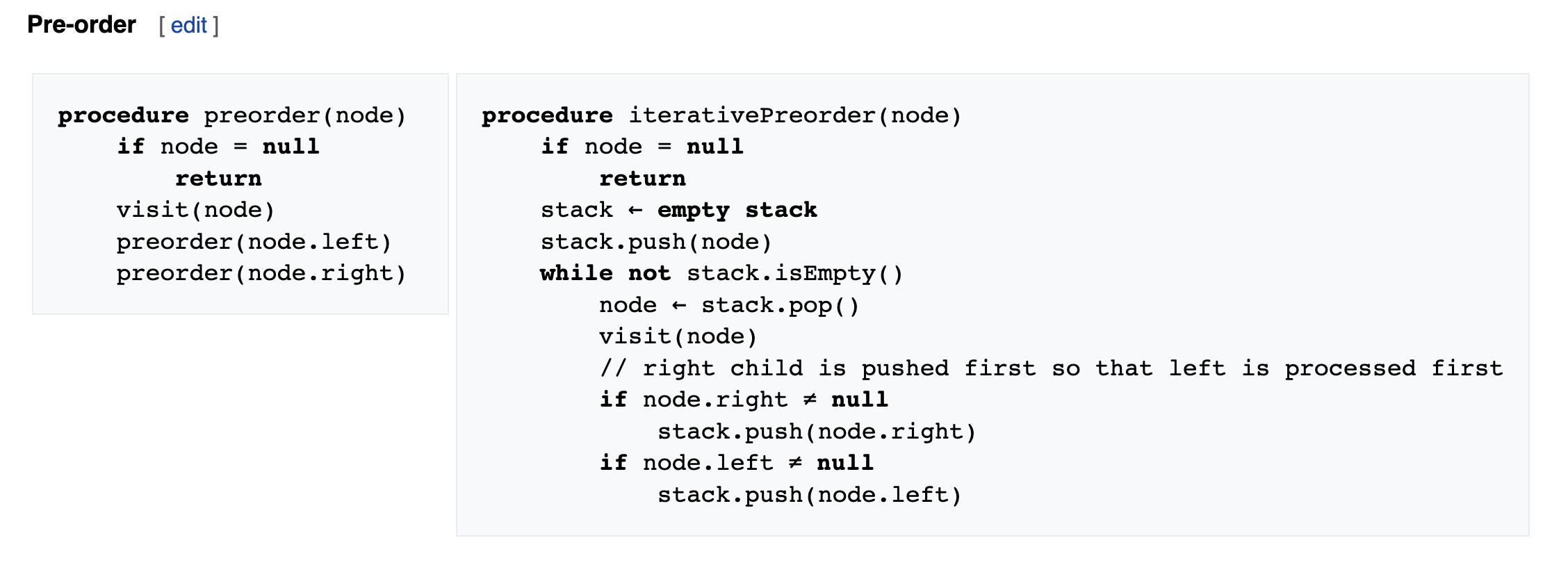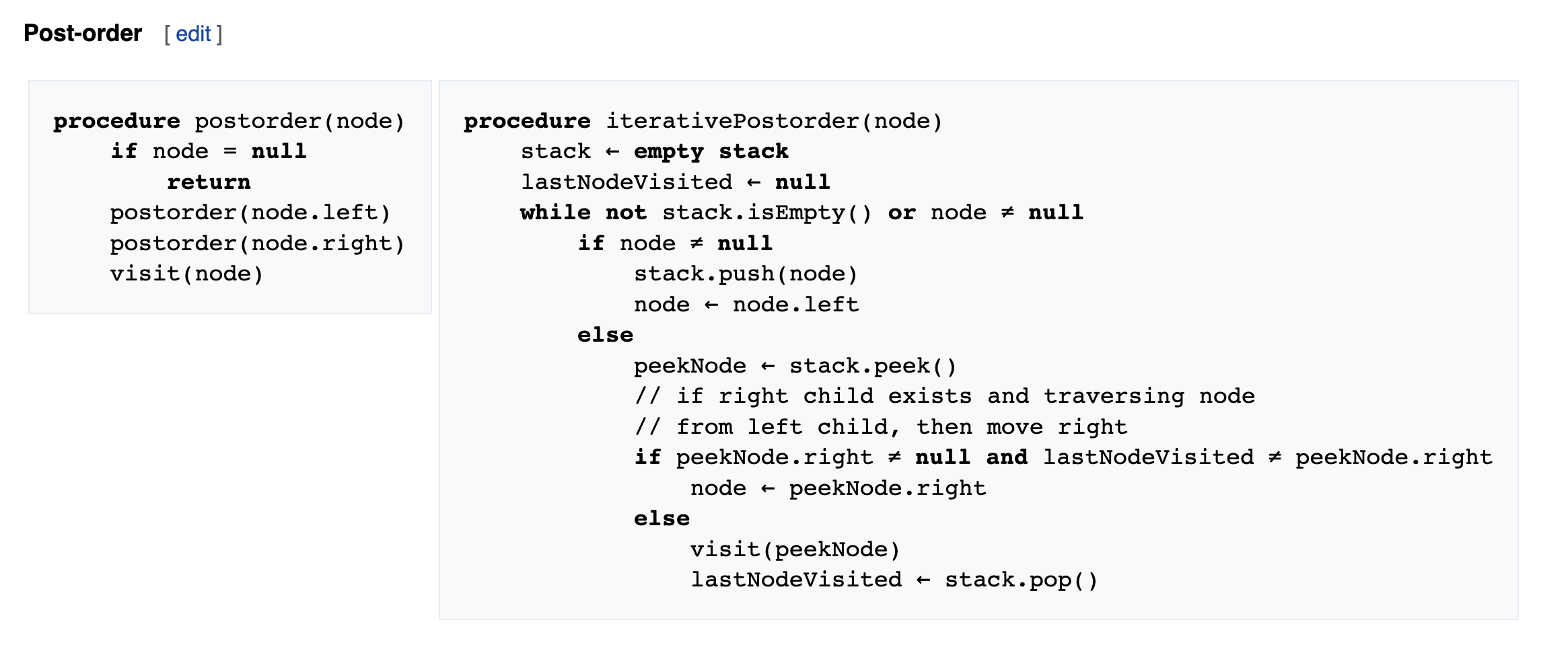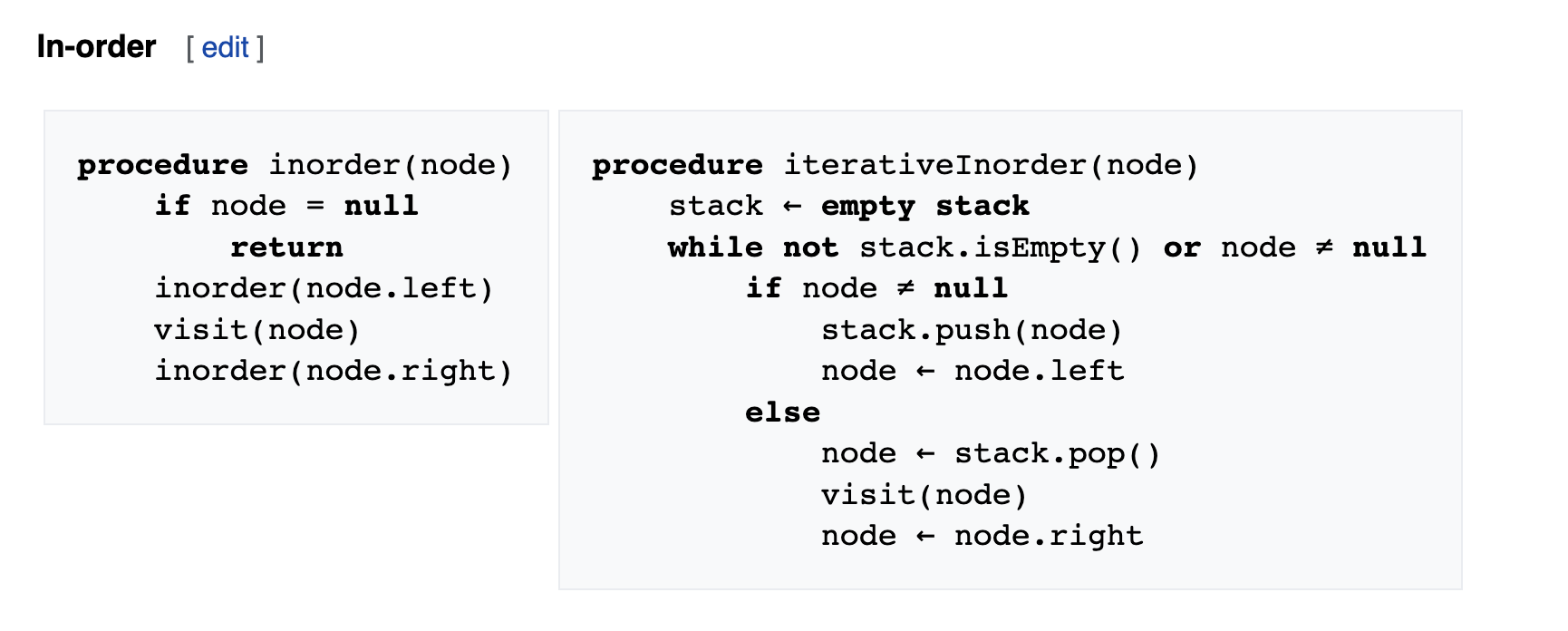# Tree Traversal §

Unlike linked lists, one-dimensional arrays and other linear data structures, which are canonically traversed in linear order, trees may be traversed in multiple ways. They may be traversed in depth-first or breadth-first order.

There are different ways to traverse a tree:

1. pre-order traversal
2. post-order traversal
3. in-order traversalDepth-first traversal (dotted path) of a binary tree:
• Pre-order (node visited at position red ●):
F, B, A, D, C, E, G, I, H;
• In-order (node visited at position green ●):
A, B, C, D, E, F, G, H, I;
• Post-order (node visited at position blue ●):
A, C, E, D, B, H, I, G, F.

Pre-order, NLR

1. Visit the current node (in the figure: position red).
2. Recursively traverse the current node’s left subtree.
3. Recursively traverse the current node’s right subtree.Post-order, LRN

1. Recursively traverse the current node’s left subtree.
2. Recursively traverse the current node’s right subtree.
3. Visit the current node (in the figure: position blue).In-order, LNR

1. Recursively traverse the current node’s left subtree.
2. Visit the current node (in the figure: position green).
3. Recursively traverse the current node’s right subtree